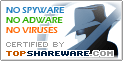# Function Grapher

SNAPSHOT

Graph maker to create 2d, 2.5d and 3D function graphs and animations.

Windows AllPlatform :
USD \$35Price :
2.77 MBFile Size :
ScreenshotScreenshot :Popularity :Function Grapher is graph maker to create 2D, 2.5D and 3D function graphs, animations and table graphs. 2D and 2.5D function graphs can be plotted in Cartesian and polar coordinate systems, and 3D function graphs can be plotted in Cartesian,cylindrical and spherical coordinate systems.

• Equation Grapher
Equation grapher is an easy-to-use software for 2D function graphing.
• Visual Matrix
Matrix calculator for matrix operation and matrix decomposition.
• Visual Data
Scientific surface data and volume data visualization software.
• Visual Probability
Compute and graph PDF, CDF and quantile values of ten more distributions.